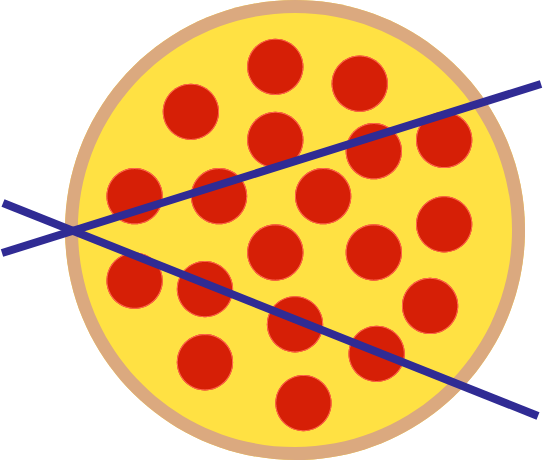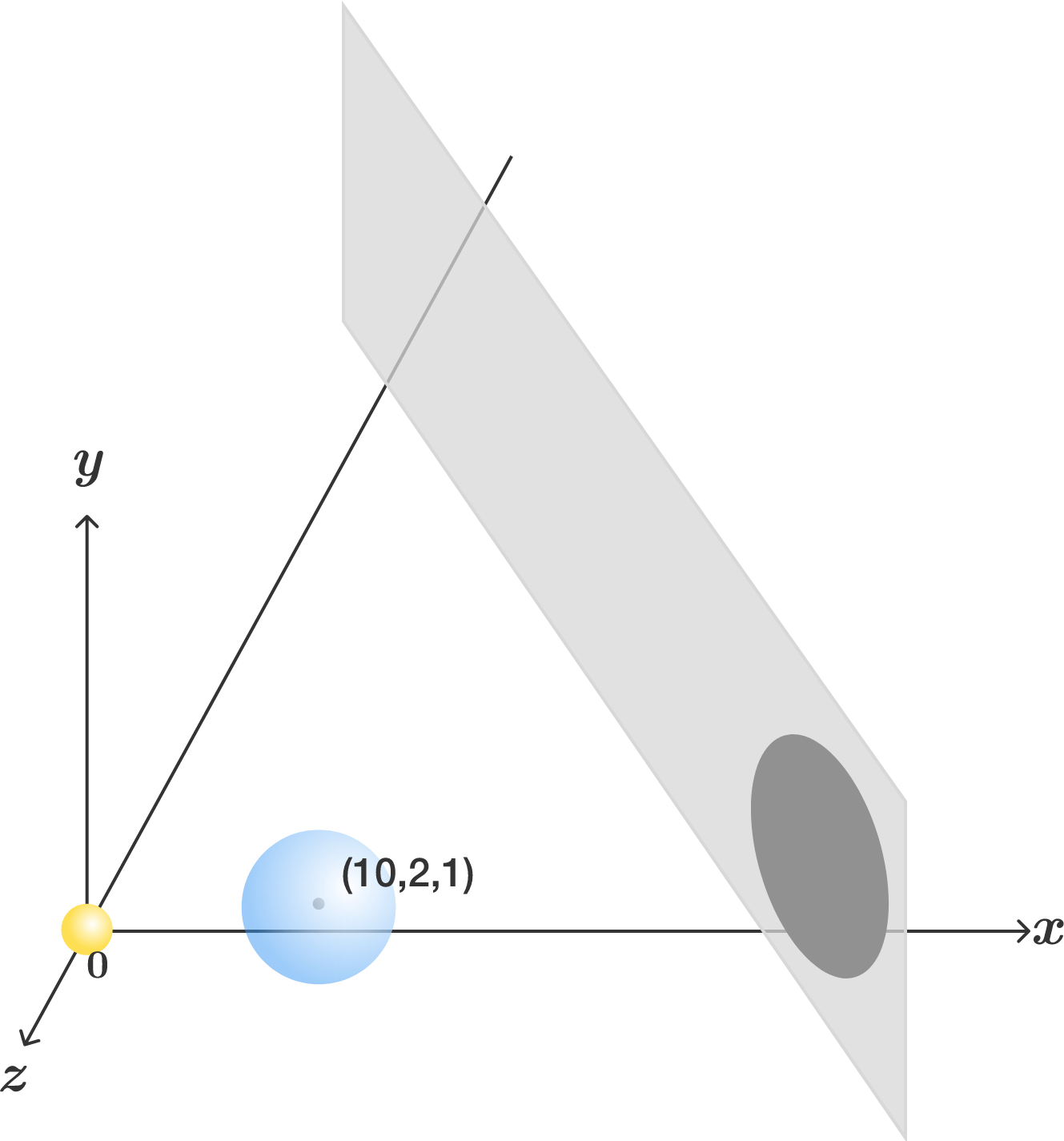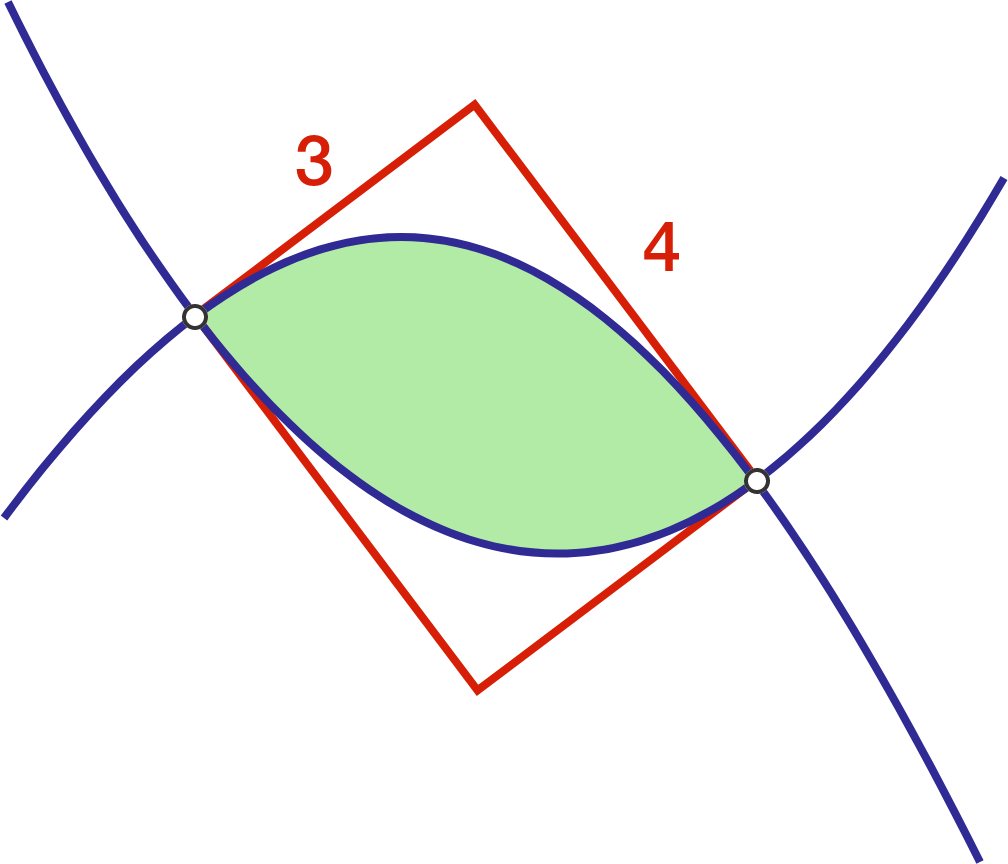# Problems of the Week

Contribute a problem3 people have each been assigned $H$ or $T$ on their foreheads, based on the results of tossing a fair coin. Each member can see each others' letters but not their own. Their common goal is to win a game with the following rules:

1. They must all make a statement at the same time, and no communication is allowed beforehand.
2. Each member's statement can either be a guess of their own letter ("H" or "T") or "pass".
3. They win if at least one person guesses correctly and no one guesses incorrectly.

With everyone applying the optimal strategy, what is their probability of winning?

Let $f : \mathbb{Z}^+ \longrightarrow \mathbb{Z}^*$ the function that assigns to each positive integer $n$ the number of rational numbers $\frac{p}{q}$ such that $\left\lbrace \begin{array}{ccc} p+q = n \\ 0<\frac{p}{q}<1 \\ \gcd(p,q)=1. \end{array}\right.$ For example, when $n=8,$ we have $2$ such rational numbers: $\frac{1}{7}$ and $\frac{3}{5}$. Hence $f(8)=2$.

What is the first positive integer $m$ such that there is no solution to $f(n)=m?$

As shown in the diagram, using 2 straight cuts from the same starting point on the perimeter, I've cut a circular pizza into 3 pieces of equal areas. However, each of the 2 identical pieces at the top and bottom has more crust than the middle piece!

A slice with larger crust has $X$% more crust than the slice with smaller crust.

Assuming the crust of the pizza is 1-dimensional, find $X$ to the nearest integer.In a Cartesian coordinate system, there are 2 spheres and 1 plane described as follows:

• $S_1:$ Centered at the origin with radius 1.
• $S_2:$ Centered at $(10,2,1)$ with radius 3.
• $P:$ Defined by the equation $9x-10z=260.$

The first sphere $S_1$ acts as a light source, the second sphere $S_2$ is opaque, and the plane $P$ is a screen.

What is the area of the umbra (the darkest part of the shadow, where an observer cannot see the light source at all) on the screen?Two parabolas (in blue) intersect at two points. The four tangent lines (in red) at their points of intersection form a $3\times 4$ rectangle. Find the area of the closed region created by both parabolas.Find the area of the region bounded by the 2 curves.

×